Next: 2-dimensional locally connected continua Up: Cyclic examples of locally Previous: Sierpinski carpet

## Menger universal curve

The Menger universal curve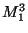is the subset of the unit cube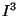whose projections onto faces of the cube are the Sierpinski carpets, i.e.,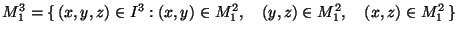[Menger 1926]. Other descriptions can be found in 1.4.1 (see also [Mayer et al. 1986, pp. 5-6, 8-9]). See Figure A.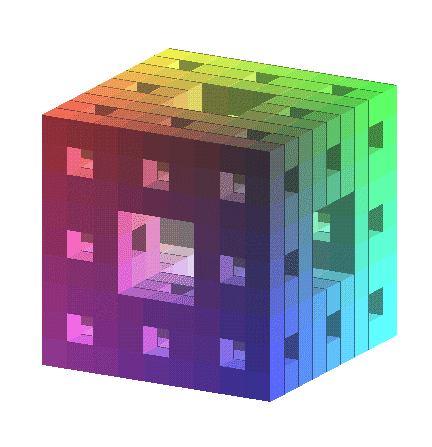1. The following statements are equivalent:
1.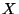is homeomorphic to;
2.is a locally connected curve with no local cut points and no planar open nonempty subsets [Anderson 1958, Theorem XII, p. 13];
3.is a homogeneous locally connected curve different from a simple close curve [Anderson 1958, Theorem XIII, p. 14];
4.is a locally connected curve with the disjoint arcs property (see Property 3 in 1.4.1);
5.is a locally connected curve and each arc inis approximately non-locally-separating arc and has empty interior in[Krupski et al. XXXXa, Theorem 3, p. 86].

2.is universal in the class of all metric separable spaces of dimension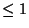[Menger 1926].

3. Z-setsZ-set incoincide with non-locally-separating closed subsets of.

If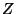is a separable metric space of dimension, thencan be embedded as a non-locally-separating subset of[Mayer et al. 1986, Theorem 6.1, p. 42].

4. Ifis a closed subset of a metric space,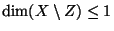and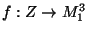is a continuous mapping with non-locally separating image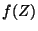, then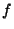can be extended to a map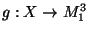such that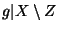is an embedding into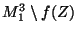[Mayer et al. 1986, Theorem 6.4, p. 44].

5. Every continuous surjection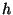between non-locally separating closed subsets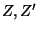ofextends to a mapping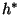ofsuch that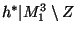is a homeomorphism onto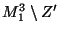[Mayer et al. 1986, Corollary 6.5, p. 44]; moreover, for every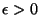there exists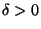such that ifis a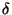-homeomorphism, thencan be taken as an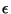-homeomorphism (see Property 4b in 1.4.1).

6. For each locally connected continuum, there exist open surjections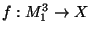and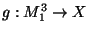such that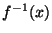is homeomorphic toand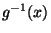is a Cantor set, for any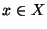[Wilson 1972].

7. Any compact 0-dimensional group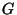acts freely onso that the orbit space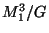is homeomorphic to[Anderson 1957, Theorem 1].

8. If a locally compact spacecontains a topological copy of, then the space of all copies ofinwith the Hausdorff metricHausdorff metric is a true absolute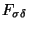-set [Krupski XXXXb].

Here you can find source files of this example.

Here you can check the table of properties of individual continua.

Here you can read Notes or write to Notes ies of individual continua.Next: 2-dimensional locally connected continua Up: Cyclic examples of locally Previous: Sierpinski carpet
Janusz J. Charatonik, Pawel Krupski and Pavel Pyrih
2001-11-30